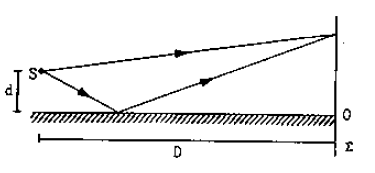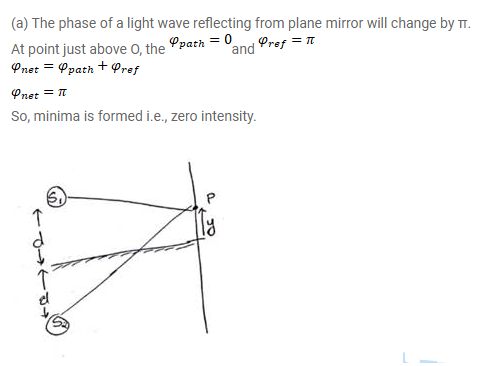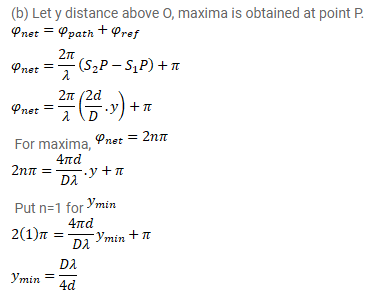# A narrow slit S transmitting light of wavelength`
Question:

A narrow slit $S$ transmitting light of wavelength $\lambda$ is placed a distance $d$ above a large plane mirror as shown in figure. The light coming directly from the slit and that coming after the reflection interface at a screen $\Sigma$ placed at a distance D from the slit.

(a) What will be the intensity at a point just above the mirror, i.e., just above 0 ?

(b) At what distance from 0 does the first maximum occur?Solution: